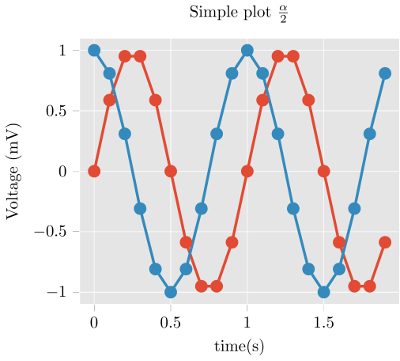Convert matplotlib figures into TikZ/PGFPlots

## Project description

The artist formerly known as matplotlib2tikz.

This is tikzplotlib, a Python tool for converting matplotlib figures into PGFPlots (PGF/TikZ) figures likefor native inclusion into LaTeX or ConTeXt documents.

The output of tikzplotlib is in PGFPlots, a TeX library that sits on top of PGF/TikZ and describes graphs in terms of axes, data etc. Consequently, the output of tikzplotlib

• can be more easily understood, and
• is more easily editable

than raw TikZ output. For example, the matplotlib figure

import matplotlib.pyplot as plt
import numpy as np

plt.style.use("ggplot")

t = np.arange(0.0, 2.0, 0.1)
s = np.sin(2 * np.pi * t)
s2 = np.cos(2 * np.pi * t)
plt.plot(t, s, "o-", lw=4.1)
plt.plot(t, s2, "o-", lw=4.1)
plt.xlabel("time (s)")
plt.ylabel("Voltage (mV)")
plt.title("Simple plot $\\frac{\\alpha}{2}$")
plt.grid(True)

import tikzplotlib

tikzplotlib.save("test.tex")

import matplotlib as mpl

plt.close()
mpl.rcParams.update(mpl.rcParamsDefault)


--> (see above) gives

\begin{tikzpicture}

\definecolor{color0}{rgb}{0.886274509803922,0.290196078431373,0.2}
\definecolor{color1}{rgb}{0.203921568627451,0.541176470588235,0.741176470588235}

\begin{axis}[
axis background/.style={fill=white!89.8039215686275!black},
axis line style={white},
tick align=outside,
tick pos=left,
title={Simple plot $$\displaystyle \frac{\alpha}{2}$$},
x grid style={white},
xlabel={time (s)},
xmajorgrids,
xmin=-0.095, xmax=1.995,
xtick style={color=white!33.3333333333333!black},
y grid style={white},
ylabel={Voltage (mV)},
ymajorgrids,
ymin=-1.1, ymax=1.1,
ytick style={color=white!33.3333333333333!black}
]
\addplot [line width=1.64pt, color0, mark=*, mark size=3, mark options={solid}]
table {%
0 0
0.1 0.587785252292473
% [...]
1.9 -0.587785252292473
};
\addplot [line width=1.64pt, color1, mark=*, mark size=3, mark options={solid}]
table {%
0 1
0.1 0.809016994374947
% [...]
1.9 0.809016994374947
};
\end{axis}

\end{tikzpicture}


(Use get_tikz_code() instead of save() if you want the code as a string.)

Tweaking the plot is straightforward and can be done as part of your TeX work flow. The fantastic PGFPlots manual contains great examples of how to make your plot look even better.

Of course, not all figures produced by matplotlib can be converted without error. Notably, 3D plots don't work.

### Installation

tikzplotlib is available from the Python Package Index, so simply do

pip install tikzplotlib


to install.

### Usage

1. Generate your matplotlib plot as usual.

2. Instead of pyplot.show(), invoke tikzplotlib by

import tikzplotlib

tikzplotlib.save("mytikz.tex")
# or
tikzplotlib.save("mytikz.tex", flavor="context")


to store the TikZ file as mytikz.tex.

3. Add the contents of mytikz.tex into your TeX source code. A convenient way of doing so is via

\input{/path/to/mytikz.tex}


Also make sure that the packages for PGFPlots and proper Unicode support and are included in the header of your document:

\usepackage[utf8]{inputenc}
\usepackage{pgfplots}
\DeclareUnicodeCharacter{2212}{−}
\usepgfplotslibrary{groupplots,dateplot}
\usetikzlibrary{patterns,shapes.arrows}


or:

\setupcolors[state=start]
\usemodule[tikz]
\usemodule[pgfplots]
\usepgfplotslibrary[groupplots,dateplot]
\usetikzlibrary[patterns,shapes.arrows]
\unexpanded\def\startgroupplot{\groupplot}
\unexpanded\def\stopgroupplot{\endgroupplot}


You can also get the code via:

import tikzplotlib

tikzplotlib.Flavors.latex.preamble()
# or
tikzplotlib.Flavors.context.preamble()

4. [Optional] Clean up the figure before exporting to tikz using the clean_figure command.

import matplotlib.pyplot as plt
import numpy as np

import tikzplotlib

tikzplotlib.clean_figure()
tikzplotlib.save("test.tex")


The command will remove points that are outside the axes limits, simplify curves and reduce point density for the specified target resolution.

The feature originated from the matlab2tikz project and is adapted to matplotlib.

### Contributing

If you experience bugs, would like to contribute, have nice examples of what tikzplotlib can do, or if you are just looking for more information, then please visit tikzplotlib's GitHub page.

### Testing

tikzplotlib has automatic unit testing to make sure that the software doesn't accidentally get worse over time. In test/, a number of test cases are specified. Those run through tikzplotlib and compare the output with a previously stored reference TeX file.

To run the tests, just check out this repository and type

pytest


tikzplotlib is published under the MIT license.

## Project details

This version0.10.10.10.00.9.170.9.160.9.150.9.140.9.13 yanked0.9.120.9.110.9.100.9.90.9.80.9.70.9.60.9.50.9.40.9.30.9.20.9.10.9.00.8.70.8.60.8.50.8.40.8.30.8.20.8.10.8.0

Uploaded source
Uploaded py3